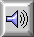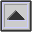PYTHAGOREAN SCALE

A series of INTERVALs devised to create a SCALE dividing the OCTAVE into more or less equal steps on the basis of powers of 2/3 or 3/2, i.e. downward or upward intervals of the FIFTH respectively. Given the series:

 2/3 1 3/2 (3/2)2 (3/2)3 (3/2)4 (3/2)5

and reducing them to intervals lying within the octave, the scale becomes:

 1 9/8 81/64 4/3 3/2 27/16 243/128 2

In this scale, the ratios between successive steps are either the whole tone 9/8 (204 cents) or the diatonic semitone 256/243 (90 cents). However, the corresponding disadvantage is that no matter how many fifths (3/2 intervals) one takes, either above or below a given note, one never arrives at an octave multiple of that note.

If the power series begun above is extended to the sixth power of (3/2) on the right and the sixth power of (2/3) on the left, then a chromatic scale of 12 tones is produced. However, as with the scale of JUST INTONATION, there is a difference between a raised semitone (SHARP) and a lowered one (FLAT). Each is above and below its related note by the interval 2187/2048 (the chromatic semitone) in the Pythagorean scale, thus making C sharp distinct from D flat, for instance.

The interval between the diatonic and chromatic semitone, which is the same as that between the sixth power of (3/2) and the exact octave, is called the Pythagorean comma and has the ratio 531441/524288.

Natural HARMONICs of a pipe or string fit more closely to a scale of just intonation than to the Pythagorean.

See: Appendix C. Compare: EQUAL TEMPERAMENT, INTONATION, TEMPERED TUNING.Sound Example: Pythagorean scale, played melodically.

Sound Example: Pythagorean scale, played as intervals.

home